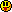﻿ Unreal Software - Thread: A* Pathfinding

# ForumCS2DScriptsA* Pathfinding

#A* Pathfinding

8 replies
Goto Page1Mami Tomoe
User
OfflineHello, for a while now I've been trying to understand how the A* path finding works.

I've taken a look at this but I couldn't figure out how to implement it into CS2D.

What I need is simple, a function that receives 2 tables, from and to.

The function will return a table with inner tables each one being a step in tiles from the from table, to the to table.

Example input:
{ 1, 1 }, { 4, 1 }

Example output:
Code:
1
2
3
4
5
6
{
{ 1, 1 }, -- Start
{ 2, 1 }, -- Path
{ 3, 1 }, -- Path
{ 4, 1 }  -- End
}

Can anyone could instruct me on how to do it, show me an example or tell me of a better way to achieve this?

Thanks.
Is death truly a bad thing? If it is, then why do we all get to experience it? And... Why does it take so long? - What is the meaning of death?
Marcell
Super User
OfflineLuaJIT and LanesUser
OfflineSpawn bots invisible on your from location and let them run around the map and let them figure it out for you until they reached your to!ai_gotoYou can always give them more movement speed if you like to make your algorithm faster. You probably could further shortcut withai_freeline
Share time limited free games hereMami Tomoe
User
Offline@Marcell, is there no way to do that without LuaJIT?
@Bowlinghead, that is actually kinda funky, I like it...
Is death truly a bad thing? If it is, then why do we all get to experience it? And... Why does it take so long? - What is the meaning of death?
Mami Tomoe
User
Offline@The Dark Shadow: Seems nice, I would spend the time trying to "steal" the code from it, but before I do, does anyone have another idea?
Is death truly a bad thing? If it is, then why do we all get to experience it? And... Why does it take so long? - What is the meaning of death?
Starkkz
Moderator
OfflineYou:Start on a point and have a goal.Have a list of nodes that you can walk over.Have a list of nodes that you can't walk over.Have G the distance you have walked from the start point to every node.Have H the euclidean distance from every node to the goal point.Have F=G+H.Have your current (walking) node being the node in the list of nodes that you can walk over with the lowest F.Have added closest nodes (that aren't on the list of nodes you can't walk over) to your current (walking) node, to the list of nodes you can walk over.Have every node you have walked over in the list of nodes you can't walk over.Have checked that your current (walking) node is the goal node.

Code:
1
2
3
4
5
6
7
8
9
10
11
12
13
14
15
16
17
18
19
20
21
22
23
24
25
26
27
28
29
30
31
32
33
34
35
36
37
38
39
40
41
42
43
44
45
46
47
48
49
50
51
52
53
54
55
56
57
58
59
60
61
62
63
64
65
66
67
68
69
70
71
72
73
74
75
76
77
78
79
80
81
82
83
84
85
86
87
88
89
90
91
92
93
94
95
96
97
98
99
100
101
102
103
104
105
106
107
108
109
110
111
112
113
114
115
116
117
118
119
120
121
122
123
124
125
126
127
128
129
130
131
132
133
134
135
136
137
138
139
140
141
142
143
144
145
146
147
148
149
150
151
152
function PathMap(x,y)
if tile(x,y,"walkable") then
return 0
end
return 1
end

function MapTeleporter(x,y)
if entity(x, y, "exists") and entity(x, y, "typename") == "Func_Teleport" then
if entity(x, y, "state") then
return entity(x, y, "int0"), entity(x, y, "int1")
end
end
end

function FindPath(fx,fy,tx,ty,Map)
if Map == nil then Map = PathMap end

if Map(fx,fy) == 1 or Map(tx,ty) == 1 then
return nil, "The start or the goal is not walkable"
end

local Node = {}
local curbase = {x = fx,y = fy}
local openlist = {{x = fx,y = fy}}

local function Euclidean(fx,fy,tx,ty)
return math.sqrt((fx-tx)^2 + (fy-ty)^2)
end

function CreateNodeConfig(x,y,open)
if not Node[x] then Node[x] = {} end
if not Node[x][y] then
if open == nil then open = false end
local n = {
Open = open,
Closed = false,
parent = {}
}
n.G = 0
n.H = Euclidean(x,y,tx,ty)
n.F = n.H
Node[x][y] = n
end
end

CreateNodeConfig(fx,fy,1)

local function FixedPath()
local i = {x = tx,y = ty}
local path = {}
while Node[i.x][i.y].parent.x and Node[i.x][i.y].parent.y do
local parent = Node[i.x][i.y].parent
local Details = {
x = i.x,
y = i.y,
dist = math.sqrt((i.x - parent.x)^2 + (i.y - parent.y)^2)
}
table.insert(path, Details)
i = parent
end
return path, #path, -1
end

local function CheckNode(l,x,y,p)
CreateNodeConfig(x,y)
if not Node[x][y].Closed and Map(x,y) == 0 then
local t = {x = x,y = y}
if p then t.parent = p end
table.insert(l, t)

local nx, ny = MapTeleporter(x, y)
if nx and ny then
CheckNode(l, nx, ny, t)
end
end
end

local score = Node[p.x][p.y].G + math.sqrt((v.x-p.x)^2 + (v.y-p.y)^2)*32
if not Node[v.x][v.y].Open then
local pos = #openlist+1
openlist[pos] = {x = v.x,y = v.y}
Node[v.x][v.y].Open = pos
Node[v.x][v.y].parent = p
Node[v.x][v.y].G = score
Node[v.x][v.y].F = score + Node[v.x][v.y].H
elseif score <= Node[v.x][v.y].G then
Node[v.x][v.y].parent = p
Node[v.x][v.y].G = score
Node[v.x][v.y].F = Node[v.x][v.y].G + Node[v.x][v.y].H
end
end

local function LowestNode()
local lVal, lID
for k, v in pairs(openlist) do
if not lVal or Node[v.x][v.y].F < Node[lVal.x][lVal.y].F then
lVal = v
lID = k
end
end
return lVal, lID
end

local function OpenListEmpty()
for k, v in pairs(openlist) do
return false
end
return true
end

while not OpenListEmpty() do
local lW, lWID = LowestNode()

if not lW or not lWID then
return nil, "Failed to find path"
else
Node[lW.x][lW.y].Closed = true
Node[lW.x][lW.y].Open = false
openlist[lWID] = nil
curbase = lW
end

if curbase.x == tx and curbase.y == ty then
return FixedPath()
end

local NearNodes = {}
CheckNode(NearNodes,curbase.x,curbase.y+1)
CheckNode(NearNodes,curbase.x+1,curbase.y)
CheckNode(NearNodes,curbase.x,curbase.y-1)
CheckNode(NearNodes,curbase.x-1,curbase.y)
if Map(curbase.x+1,curbase.y) == 0 and Map(curbase.x,curbase.y+1) == 0 then CheckNode(NearNodes,curbase.x+1,curbase.y+1) end
if Map(curbase.x+1,curbase.y) == 0 and Map(curbase.x,curbase.y-1)== 0 then CheckNode(NearNodes,curbase.x+1,curbase.y-1) end
if Map(curbase.x-1,curbase.y) == 0 and Map(curbase.x,curbase.y+1) == 0 then CheckNode(NearNodes,curbase.x-1,curbase.y+1) end
if Map(curbase.x-1,curbase.y) == 0 and Map(curbase.x,curbase.y-1) == 0 then CheckNode(NearNodes,curbase.x-1,curbase.y-1) end
for i = 1, #NearNodes do
end
end
return nil, "Couldn't find the path"
end

function PathWalkable(path)
for k, v in pairs(path) do
if not tile(v.x,v.y,"walkable") then
return false
end
end
return true
end

The code is old so it's not optimized for the best performance.
Mami Tomoe
User
OfflineIs death truly a bad thing? If it is, then why do we all get to experience it? And... Why does it take so long? - What is the meaning of death?
DC
Offlineai_goto is internally using A* already. So if you want to control bots then this is the best option. Of course this doesn't work if you want to use it for other things like your own fully scripted characters or whatever.1